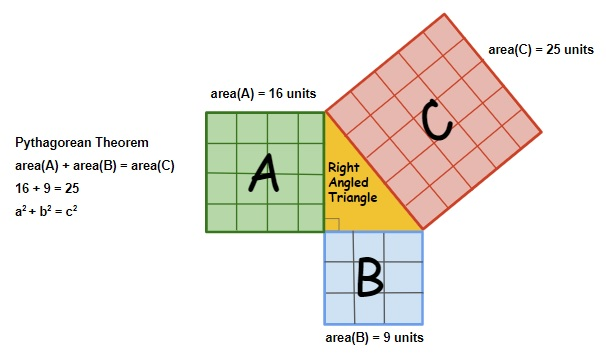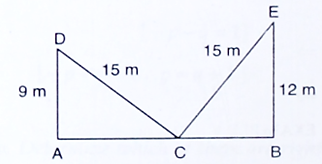# Pythagoras Theorem

## Pythagorean Theorem Formulas , Applications & Examples

Do you know it was back during the 6th century B.C that eminent Greek mathematician coined the fundamental theorem which later came to be known as Pythagorean Theorem?

He was the 1st individual to come up with this theorem. So, what does it entail? Simple – the relation between the three sides of a right-angle triangle. If we revise the definition once, Pythagorean or Pythagoras Theorem states that the summation of squares of 2 sides’ (base and height) lengths of a triangle is equivalent to the square of the 3rd side, which is the longest. This side is termed as the hypotenuse. Well, learning the Pythagoras Theorem formula and different ways to prove it are equally simple if you understand it thoroughly.

Here, at Vedantu, we aim to help you precisely with the same so that you can excel in exams not only with better marks but also with a stronger concept.

Pythagorean Theorem formula and examples

• 1. Consider a triangle which has one 90° angle

•Here, ‘a’ is the perpendicular, ‘b’ is the base and ‘c’ is the hypotenuse. You can term ‘a’ and ‘b’ as the legs of that triangle which meet each other at 90°.

Hence, the formula will be a2 + b2 = c2.

There’s another way of stating this theorem.

• 2. Consider three squares mounted on the three sides of a right-angled triangle

• In this case, ‘a’ is the base, ‘b’ is the perpendicular and ‘c’ is the hypotenuse.

So, the formula will be the area of a + area of b = area of c.

Now, let’s solve a simple problem here:

If a = 6 and b = 8, then what is the value of hypotenuse, x?

Solution:

According to the Pythagoras Theorem formula, it is x2 = 62 + 82.

Now, consider it this way, x2 = 100, because 62 is 36 and 82 is 64.

So, x = , i.e., 10.

Vedantu guides thoroughly with various Pythagorean Theorem formula and examples so that students get a grip and can solve mathematical problems effortlessly. Not only students in schools but those appearing in competitive exams can also find our formulae list handy and easy to learn.

Besides, students can also learn about Pythagorean Theorem formula proof and solve simple to complex numerical calculations.

Know the real time applications of Pythagoras formula

Interestingly, there are various applications of Pythagoras Theorem in real life. Some of those are as follows:

• a) Oceanographers calculate sound speed in water using this formula.

• b) Meteorologists and aerospace scientists use it to determine the source of a sound and its range.

• c) The theorem is largely used in woodworking, architecture or such other construction projects.

• So, if you opt for such careers, rest assured you’ll need in-depth knowledge of the Pythagoras Theorem.

Applications

There are many applications of Pythagorean theorem some are listed below:

• • To determine the slope of the triangle.

• • In construction of buildings for laying out square angles and for building fences.

• • GPS

• • Oscilloscopes

• • Mechanical Engineering calculation

• • Design engineering

• • Architecture Polar coordinates

• • in math for oceanography, Trigonometry and Calculus.

• Question: In a right angled triangle, the square of the hypotenuse is equal to the sum of the squares of the other two sides.

Given: A right-angled triangle ABC in which $\angle B = 90^\circ$.

To Prove: ${\left( {{\text{Hypotenuse}}} \right)^2} = {\left( {{\text{Base}}} \right)^2} + {\left( {{\text{Perpendicular}}} \right)^2}$
i.e., $A{C^2} = A{B^2} + B{C^2}$

Construction: From B draw $BD \bot AC.$Proof: In triangles ADB and ABC, we have
$\angle ADB = \angle ABC$ [Each equal to $90^\circ$]
and, $\angle A = \angle A$ [Common]

So, by AA-similarity criterion, we have
$\Delta ADB \sim \Delta ABC$
$\Rightarrow \,\,\frac{{AD}}{{AB}} = \frac{{AB}}{{AC}}$ [$\because$ In similar triangles corresponding sides are proportional]$\Rightarrow A{B^2} = AD \times AC$ ……. (i)

In triangles BDC and ABC, we have
$\angle CDB = \angle ABC$ [Each equal to $90^\circ$]
and, $\angle C = \angle C$

So, by AA-similarity criterion, we have
$\Delta BDC \sim \Delta ABC$
$\Rightarrow \frac{{DC}}{{BC}} = \frac{{BC}}{{AC}}$ [$\because$ In similar triangles corresponding sides are proportional]
$\Rightarrow B{C^2} = AC \times DC$ ……… (ii)
Adding equations (i) and (ii), we get
$A{B^2} + B{C^2} = AD \times AC + AC \times DC$
$\Rightarrow \,\,A{B^2} + B{C^2} = AC\left( {AD + DC} \right)$
$\Rightarrow \,\,A{B^2} + B{C^2} = AC \times AC$
$\Rightarrow A{B^2} + B{C^2} = A{C^2}$
Hence, $A{C^2} = A{B^2} + B{C^2}$.

Question: A ladder 15 m long reaches a window which is 9 m above the ground on one side of a street. Keeping its foot at the same point, the ladder is turned to other side of the street to reach a window 12 m high. Find the width of the street.

Solution:

Let AB be the width of the street and C be the foot of the ladder. Let D and E be the windows at heights of 9 m and 12 m respectively from the ground. Then, CD and EF are the two positions of the ladder.

Clearly, AD = 9 m, BE = 12 m, CD = CE = 15 m.

In $\Delta \,ACD,$ we have$C{D^2} = A{C^2} + A{D^2}$
$\Rightarrow \,\,{15^2} = A{C^2} + {9^2}$
$\Rightarrow \,\,A{C^2} = 225 - 81 = 144$
$\Rightarrow \,\,AC = 12\,m$
In $\Delta \,BCE,$ we have
$C{E^2} = B{C^2} + B{E^2}$
$\Rightarrow \,\,{15^2} = B{C^2} + {12^2}$
$\Rightarrow \,\,B{C^2} = 225 - 144 = 81$
$\Rightarrow \,\,BC = 9\,m$
Hence, width of the street $= AB = AC + CB = \left( {12 + 9} \right)m = 21\,m$.

Question: A right triangle has hypotenuse of length p cm and one side of length q cm. If $p - q = 1$, find the length of the third side of the triangle.

Options:
(a) $\sqrt {q + 1}$
(b) $\sqrt {q - 1}$
(c) $\sqrt {2q + 1}$
(d) $\sqrt {2q - 1}$

Solution:
Let the third side be x cm. Then, by Pythagoras theorem, we have
${p^2} = {q^2} + {x^2}$
$\Rightarrow {x^2} = {p^2} - {q^2} = \left( {p - q} \right)\left( {p + q} \right) = p + q$ $\left[ {\because \,\,p - q = 1} \right]$
$\Rightarrow x = \sqrt {p + q} = \sqrt {2q + 1}$ $\left[ {\because \,\,p - q = 1\,\,\,\therefore \,\,p = q + 1} \right]$

Hence, the length of the third side is $\sqrt {2q + 1}$ cm.

What to expect from Vedantu?

Being one of the most reliable live online tutoring platforms in India, Vedantu offers class-specific comprehensive solutions.

• • Students can easily download Pythagorean Theorem formula derivation and more in PDF format from Vedantu’s website.

• • They can access ample study material, sample papers, revision notes, etc. according to their board and syllabus.

• • Leaners can also study and clarify their confusions from subject-matter experts, right from the comfort of their home.

• Besides, Vedantu also brings NCERT solutions, RS Aggarwal solutions, RD Sharma solutions and more to strengthen the concept of Pythagoras Theorem formula further. You can learn at your own pace with optimum support from us. So, fortify your base today to create a successful career tomorrow.